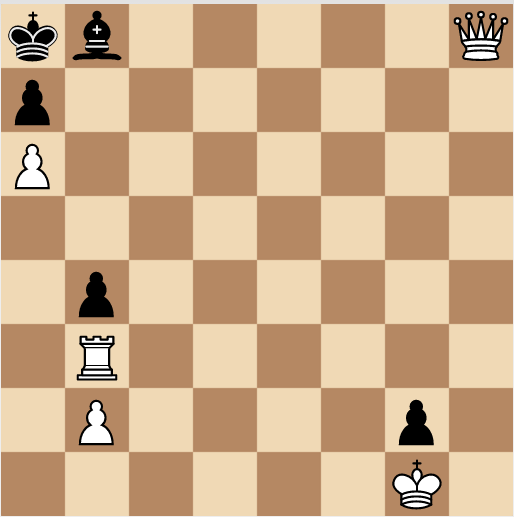# Mate In 3

It is White's move. What must White play in order to mate in 3?The bottom left corner square has coordinates $(1, 1)$. Each piece has a value: $\text{Queen}=10$, $\text{Rook}=5$, $\text{Pawn}=1$ and $\text{King}=4$.

Submit your answer as $V\times { x }_{ 1 }\times { y }_{ 1 }$, where $V$ denotes the value of the piece that White must move and the coordinates of the square where it has to go are $\left( { x }_{ 1 },{ y }_{ 1 } \right)$.

Clarification: Black plays optimally.

×